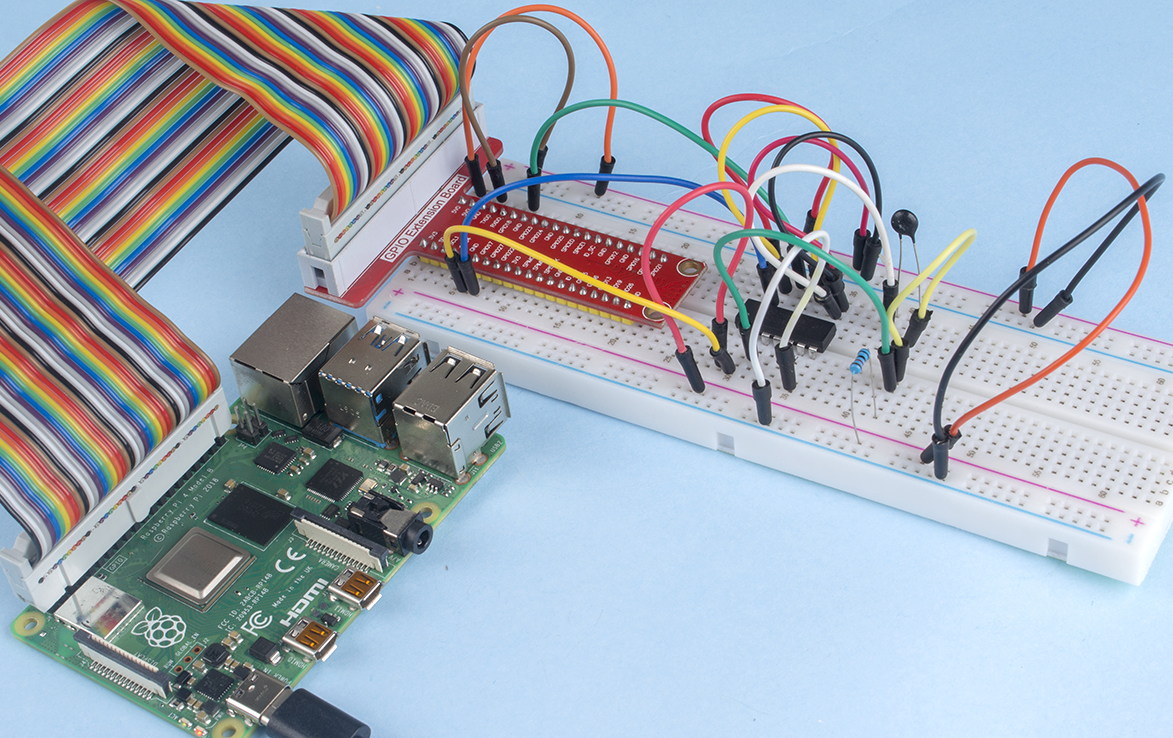# 2.2.2 Thermistor¶

## Introduction¶

Just like photoresistor can sense light, thermistor is a temperature sensitive electronic device that can be used for realizing functions of temperature control, such as making a heat alarm.

## Required Components¶

In this project, we need the following components.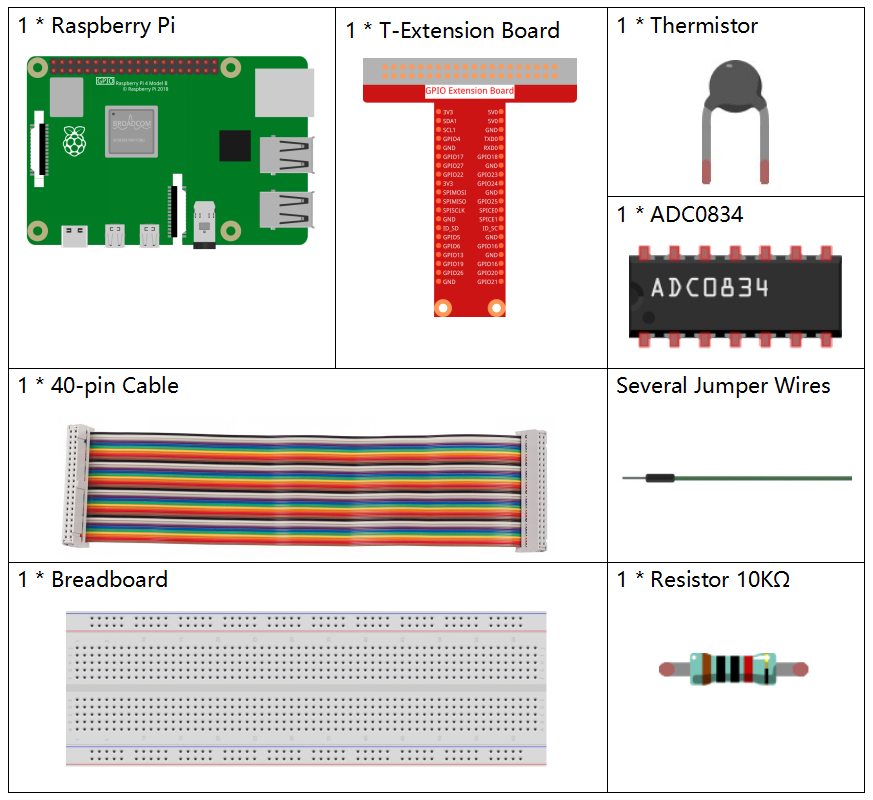Name

ITEMS IN THIS KIT

Raphael Kit

337

Raphael Kit

COMPONENT INTRODUCTION

GPIO Extension Board

Jumper Wires

Resistor

Thermistor

-

## Schematic Diagram¶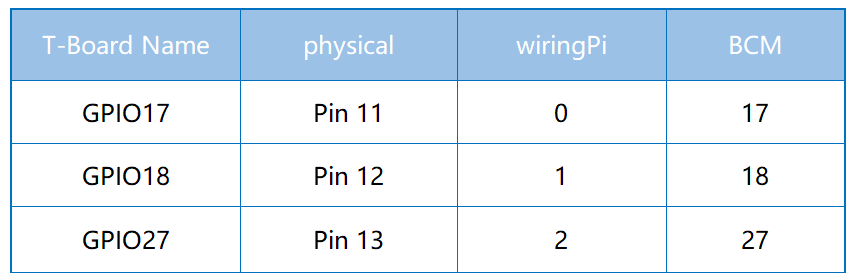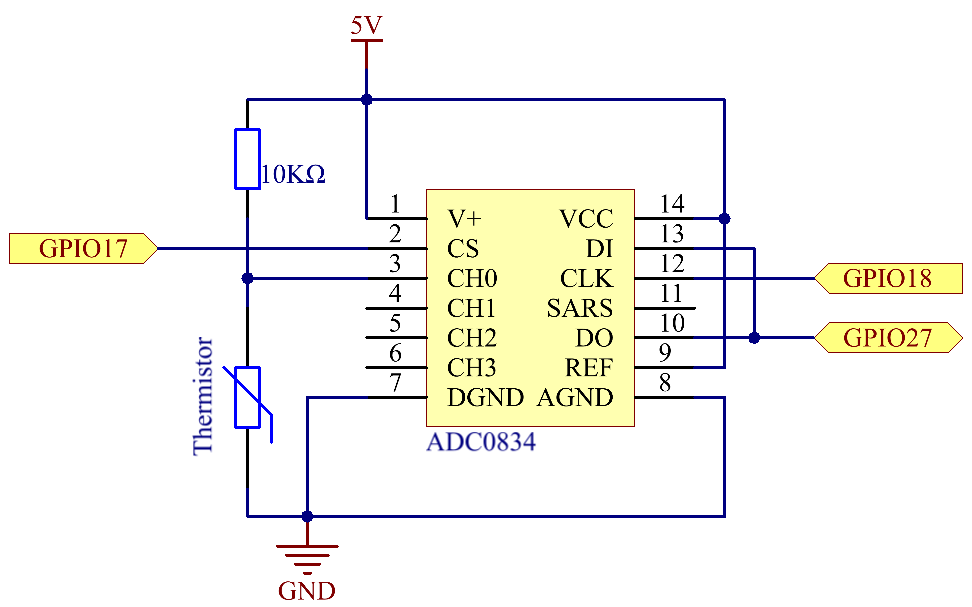## Experimental Procedures¶

Step 1: Build the circuit.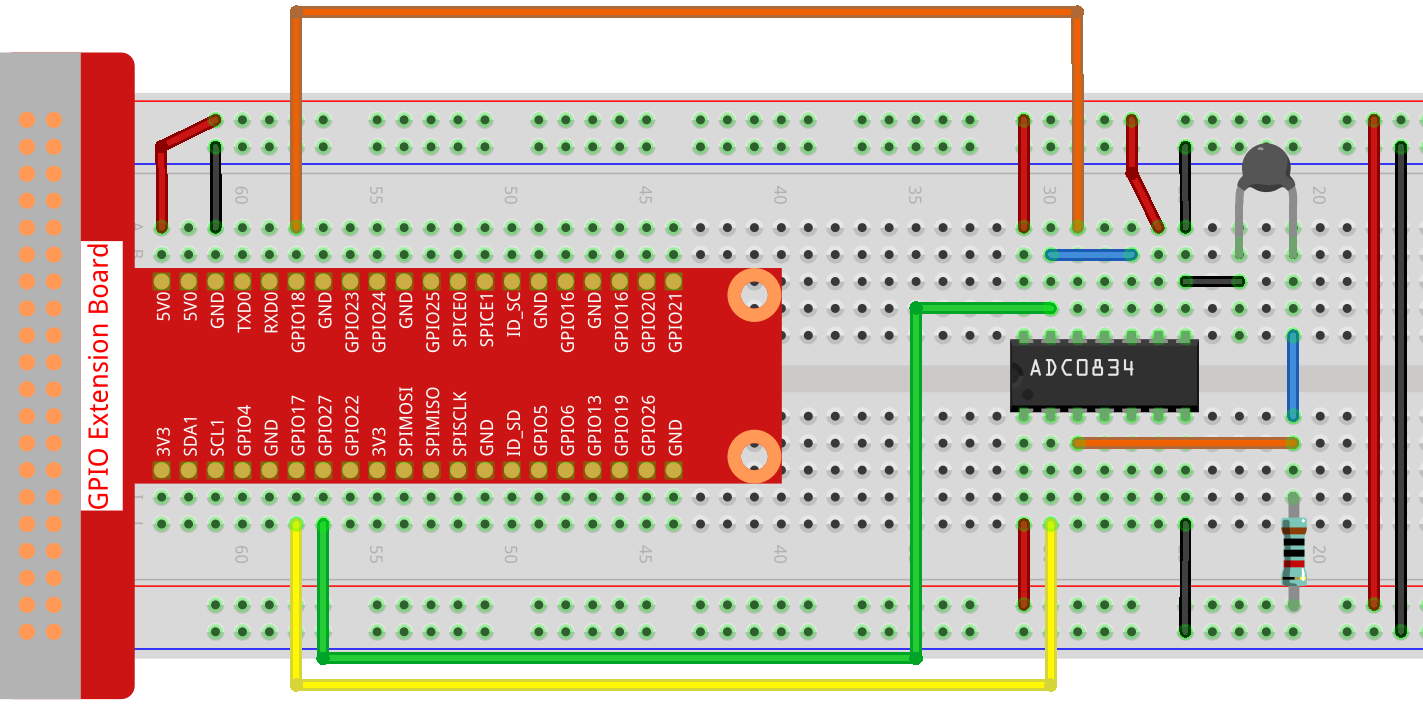Step 2: Go to the folder of the code.

```cd ~/raphael-kit/nodejs/
```

Step 3: Run the code.

```sudo node thermistor.js
```

With the code run, the thermistor detects ambient temperature which will be printed on the screen once it finishes the program calculation.

Code

```const Gpio = require('pigpio').Gpio;

setInterval(() => {
var Vr = 5 * value / 255;
var Rt = 10000 * Vr / (5 - Vr);
var temp = 1 / ((Math.log(Rt/10000) / 3950)+(1 / (273.15 + 25)));
var cel = (temp - 273.15).toFixed(2);
var Fah = (cel * 1.8 + 32).toFixed(2);
console.log(`Celsius: \${cel} C  Fahrenheit: \${Fah} F\n`);
}, (error)=>{
console.log("Error: " + error);
});
}, 1000);
```

Code Explanation

```setInterval(() => {
var Vr = 5 * value / 255;
var Rt = 10000 * Vr / (5 - Vr);
var temp = 1 / ((Math.log(Rt/10000) / 3950)+(1 / (273.15 + 25)));
var cel = (temp - 273.15).toFixed(2);
var Fah = (cel * 1.8 + 32).toFixed(2);
console.log(`Celsius: \${cel} C  Fahrenheit: \${Fah} F\n`);
}, (error)=>{
console.log("Error: " + error);
});
}, 1000);
```

We can read the `value` of the thermistor through the statement `adc.read(0).then((value) => {...})`

```var Vr = 5 * value / 255;
var Rt = 10000 * Vr / (5 - Vr);
var temp = 1 / ((Math.log(Rt/10000) / 3950)+(1 / (273.15 + 25)));
var cel = (temp - 273.15).toFixed(2);
var Fah = (cel * 1.8 + 32).toFixed(2);
console.log(`Celsius: \${cel} C  Fahrenheit: \${Fah} F\n`);
```

These operations convert the thermistor value to a Celsius temperature value.

```var Vr = 5 * value / 255;
var Rt = 10000 * Vr / (5 - Vr);
```

These two lines of code are used to calculate the voltage distribution from the read values, resulting in Rt (resistance of the thermistor).

```var temp = 1 / ((Math.log(Rt/10000) / 3950)+(1 / (273.15 + 25)));
```

This code refers to substituting Rt into the formula TK=1/(ln(RT/RN)/B+1/TN) to get the temperature in Kelvin.

```var cel = (temp - 273.15).toFixed(2);
```

This paragraph is to convert the Kelvin temperature to Celsius with two decimal places.

```var Fah = (cel * 1.8 + 32).toFixed(2);
```

This paragraph converts Celsius to Fahrenheit with two decimal places.

```console.log(`Celsius: \${cel} C  Fahrenheit: \${Fah} F\n`);
```

Print Celsius, Fahrenheit and their units on the terminal.

## Phenomenon Picture¶